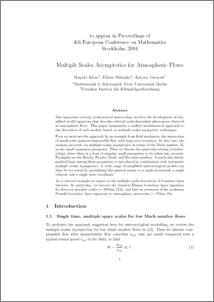Repository: Freie Universität Berlin, Math Department

# Multiple Scales Asymptotics for Atmospheric Flows

Klein, R. and Mikusky, E. and Owinoh, A.Z. (2004) Multiple Scales Asymptotics for Atmospheric Flows. Proceedings of the 4th European Conference on Mathematics .Preview

278kB

## Abstract

One important activity of theoretical meteorology involves the development of simplified model equations that describe selected scale-dependent phenomena observed in atmospheric flows. This paper summarizes a unified mathematical approach to the derivation of such models, based on multiple scales asymptotic techniques. First we motivate the approach by an example from fluid mechanics, the interaction of small-scale quasi incompressible flow with long-wave acoustics. In this case, the analysis proceeds via multiple scales asymptotics in terms of the Mach number, M, as the small expansion parameter. Then we discuss the particular setting of meteorology, where there is a host of singular small parameters to be taken into account. Examples are the Rossby, Froude, Mach, and Strouhal numbers. A particular distinguished limit among these parameters is introduced in combination with systematic multiple scales asymptotics. A wide range of simplified meteorological models can then be recovered by specializing this general ansatz to a single horizontal, a single vertical, and a single time coordinate. As a concrete example we report on the multiple scales derivation of boundary layer theories. In particular, we recover the classical Ekman boundary layer equations for flows on synoptic scales (∼ 500 km, 12 h), and find an extension of the nonlinear Prandtl boundary layer equations to atmospheric mesoscales (∼ 70 km,2h).

Item Type: Article Mathematical and Computer Sciences > Mathematics Department of Mathematics and Computer Science > Institute of Mathematics > Geophysical Fluid Dynamics Group 471 Ulrike Eickers 24 Jun 2009 13:45 03 Mar 2017 14:40

Repository Staff Only: item control page